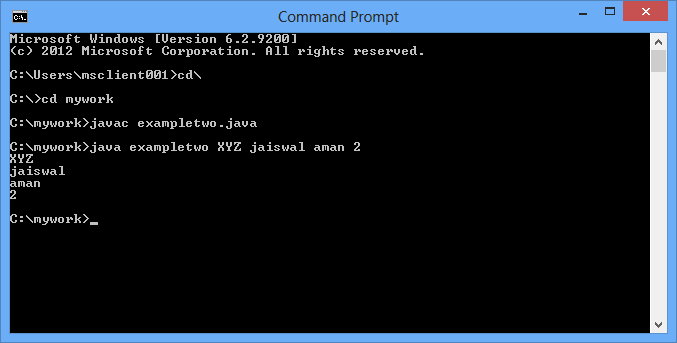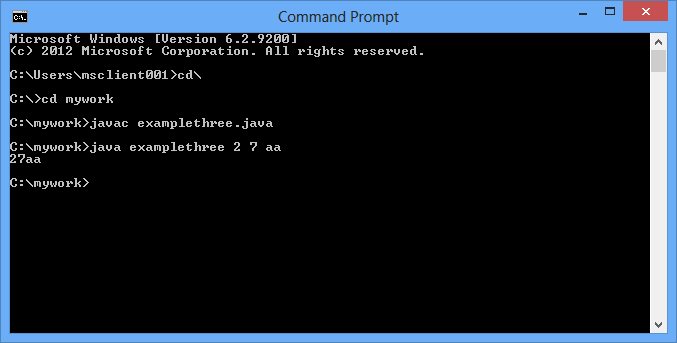The java command-line argument is an argument i.e. passed at the time of running the java program.

The arguments passed from the console can be received in the java program and it can be used as an input.

So, it provides a convenient way to check the behavior of the program for the different values. You can pass N (1, 2, 3 and so on) numbers of arguments from the command prompt.

Let us see it through some examples:

1)      In this example, we are receiving only one argument and printing it. To run this java program, you must pass at least one argument from the command prompt.

`    class exampleone{      public static void main(String args[]){      System.out.println("Your first argument is: "+args);      }      }  `

compile by > javac exampleone.java

run by > java exampleone firstvalue

Output: Your first argument is: firstvalue

2)      In this example, we are printing all the arguments passed from the command-line. For this purpose, we have traversed the array using for loop.

`    class exampletwo{      public static void main(String args[]){            for(int i=0;i<args.length;i++)      System.out.println(args[i]);            }      }  `compile by > javac exampletwo.java

run by > java exampletwo XYZ jaiswal aman 2

##### Output:

XYZ

jaiswal

aman

3)     In this example, we are going to print the sum (concatination) of the strings passed through command line. To run this java program, you must pass at least one argument from the command prompt and we have traversed the array using for loop.

`public class examplethree{public static void main(String args[]){String sum="";for(int i=0;i<args.length;i++){sum=sum+args[i];}System.out.println(sum);}}`compile by > javac examplethree.java

run by > java examplethree 2 7 aa

##### Output:

27aa

4)    In this example, we are going to print the sum of the integer values passed through command line. To run this java program, you must pass at least one argument from the command prompt and we have traversed the array using for loop.

`public class examplefour{public static void main(String args[]){int sum=0;for(int i=0;i<args.length;i++){sum=sum+Integer.parseInt(args[i]);}System.out.println(+sum);}}`compile by > javac examplefour.java

run by > java examplefour 2 6 8

##### 16

Modified On Mar-13-2018 04:39:25 AM